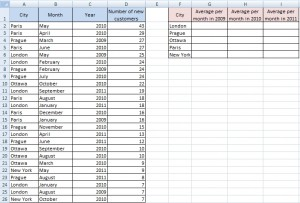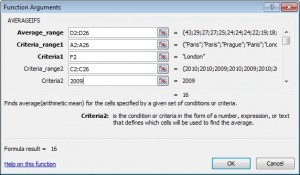# Average with multiple conditions

There is problem with average for some specific values in a table. We need to make average of the items that meet several criteria. Following table represents data of some worldwide company. You can see branch offices in the cities and number of their new customers per each month and year.How to do average of specific branch offices for specific year?

## Solution for Excel 2007

For version 2007 is very easy to calculate this. Just use AVERAGEIFS function. Formula for average of London in 2009 is like this:

`=AVERAGEIFS(D2:D26,A2:A26,F2,C2:C26,2009)`If you need this to copy to the other items, you have to fill \$ character into the formula. Be careful, \$ isn’t in each argument.
`=AVERAGEIFS(\$D\$2:\$D\$26,\$A\$2:\$A\$26,F2,\$C\$2:\$C\$26,2009)`

## Solution for Excel 2003

In version 2003 is AVERAGEIFS function missing. Therefore we have to use array formula:

`{=AVERAGE(IF((A2:A26=F2)*(C2:C26=2009),D2:D26))}`

Explanation:

(A2:A26=F2)*(C2:C26=2009) are conditions. To add more criteria continue with: * (another criterion)* (another criterion)…

(A2:A26=F2) means: choose only London value. (C2:C26=2009) means:  choose only year 2009.

As in the previous example, If you need this to copy to the other items, you have to fill \$ character into the formula.

`{=AVERAGE(IF((\$A\$2:\$A\$26=F2)*(\$C\$2:\$C\$26=2009),\$D\$2:\$D\$26))}`

And the result is:#DIV/0! error means that there is no values to average.

1.